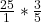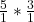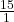## What is the product of 3/5 and 25. Is the product more or less than 14? Explain your answer in complete sentences. (3/5 is a fraction

Question

What is the product of 3/5 and 25. Is the product more or less than 14? Explain your answer in complete sentences.
(3/5 is a fraction Not Disvison )

in progress 0
2 months 2021-07-25T05:18:06+00:00 1 Answers 2 views 0

15, The answer is greater than 14 because when you multiply the 2 fractions you get a number that is greater than 15

Step 1: Set up equationStep 2: Cross reduce

you can cross reduce 25 and 5 because they are both share a common divisorStep 3: Multiply numerator and denominator together